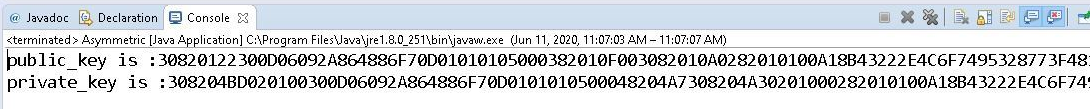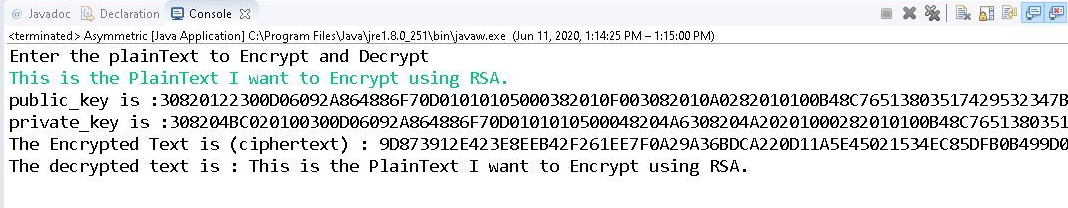GeeksforGeeks App
Open AppBrowser
Continue

# Asymmetric Encryption Cryptography in Java

Cryptography is the study of different techniques to secure data from an unauthorized entity. In computer science, we try to develop strategies and practices for protecting sensitive data. Most of the cryptography involves very advanced Mathematical functions used for securing data. The sole purpose of the algorithms developed for cryptography is to hide data from the attacker or middleman.
In the previous article, we have studied the different methods, classes and approach to perform the symmetric encryption and decryption. In this article, we will understand asymmetric encryption.
Asymmetric Encryption also called as private/public key Encryption is a mathematical relation between two keys, one for encryption and the other for decryption. For example, if there are two keys “K1” and “K2”, then if key “K1” is used for encryption and “K2” is used for decryption. If “K1” is used for decryption, then “K2” is used for encryption.

Before implementing the asymmetric encryption using the RSA algorithm, we will first see how to generate a keypair(public, private). The following steps can be followed in order to generate asymmetric key:

• We need to first generate public & private key using the SecureRandom class. SecureRandom class is used to generate random number.

SecureRandom random = new SecureRandom();

• The KeyGenerator class will provide getInstance() method which can be used to pass a string variable which denotes the Key Generation Algorithm. It returns KeyGenerator Object. We are using RSA algorithm for generating the keys.

KeyPairGenerator KPGenerator = KeyPairGenerator.getInstance(Key_Generation_Algorithm_string_variable);

• Initializing the keyGenerator object with 2048 bits key size and passing the random number.

keyPairGenerator.initialize(2048, secureRandom);

• Now, the secret key is generated and if we wish to actually see the generated key which is an object, we can convert it into hexbinary format using DatatypeConverter.

Below is the implementation of the above approach:

## Java

 `// Java program to create a``// asymmetric key` `package` `java_cryptography;``import` `java.security.KeyPair;``import` `java.security``    ``.KeyPairGenerator;``import` `java.security``    ``.SecureRandom;``import` `javax.xml.bind``    ``.DatatypeConverter;` `// Class to create an asymmetric key``public` `class` `Asymmetric {` `    ``private` `static` `final` `String RSA``        ``= ``"RSA"``;` `    ``// Generating public and private keys``    ``// using RSA algorithm.``    ``public` `static` `KeyPair generateRSAKkeyPair()``        ``throws` `Exception``    ``{``        ``SecureRandom secureRandom``            ``= ``new` `SecureRandom();` `        ``KeyPairGenerator keyPairGenerator``            ``= KeyPairGenerator.getInstance(RSA);` `        ``keyPairGenerator.initialize(``            ``2048``, secureRandom);` `        ``return` `keyPairGenerator``            ``.generateKeyPair();``    ``}` `    ``// Driver code``    ``public` `static` `void` `main(String args[])``        ``throws` `Exception``    ``{``        ``KeyPair keypair``            ``= generateRSAKkeyPair();` `        ``System.out.println(``            ``"Public Key is: "``            ``+ DatatypeConverter.printHexBinary(``                  ``keypair.getPublic().getEncoded()));` `        ``System.out.println(``            ``"Private Key is: "``            ``+ DatatypeConverter.printHexBinary(``                  ``keypair.getPrivate().getEncoded()));``    ``}``}`

Output:Encryption and Decryption using the asymmetric key: In the above steps, we have created the public & private keys for Encryption and Decryption. Now, let us implement Asymmetric Encryption using the RSA algorithm. The following steps can be followed in order to implement the encryption and decryption.

• The cipher class is used for two different modes the encryption and decryption. As Asymmetric encryption uses different keys, we use the private key for encryption and the public key for decryption.

cipher.init(Cipher.ENCRYPT_MODE, privateKey);
cipher.init(Cipher.DECRYPT_MODE, publicKey);

• The doFinal() method is invoked on cipher which encrypts/decrypts data in a single-part operation, or finishes a multiple-part operation and returns byte array.
• Finally we get the Cipher text after Encryption with ENCRYPT_MODE.

Below is the implementation of the above approach:

## Java

 `// Java program to perform the``// encryption and decryption``// using asymmetric key` `package` `java_cryptography;``import` `java.security.KeyPair;``import` `java.security.KeyPairGenerator;``import` `java.security.PrivateKey;``import` `java.security.PublicKey;``import` `java.security.SecureRandom;``import` `java.util.Scanner;` `import` `javax.crypto.Cipher;``import` `javax.xml.bind``    ``.DatatypeConverter;` `public` `class` `Asymmetric {` `    ``private` `static` `final` `String RSA``        ``= ``"RSA"``;``    ``private` `static` `Scanner sc;` `    ``// Generating public & private keys``    ``// using RSA algorithm.``    ``public` `static` `KeyPair generateRSAKkeyPair()``        ``throws` `Exception``    ``{``        ``SecureRandom secureRandom``            ``= ``new` `SecureRandom();``        ``KeyPairGenerator keyPairGenerator``            ``= KeyPairGenerator.getInstance(RSA);` `        ``keyPairGenerator.initialize(``            ``2048``, secureRandom);``        ``return` `keyPairGenerator``            ``.generateKeyPair();``    ``}` `    ``// Encryption function which converts``    ``// the plainText into a cipherText``    ``// using private Key.``    ``public` `static` `byte``[] do_RSAEncryption(``        ``String plainText,``        ``PrivateKey privateKey)``        ``throws` `Exception``    ``{``        ``Cipher cipher``            ``= Cipher.getInstance(RSA);` `        ``cipher.init(``            ``Cipher.ENCRYPT_MODE, privateKey);` `        ``return` `cipher.doFinal(``            ``plainText.getBytes());``    ``}` `    ``// Decryption function which converts``    ``// the ciphertext back to the``    ``// original plaintext.``    ``public` `static` `String do_RSADecryption(``        ``byte``[] cipherText,``        ``PublicKey publicKey)``        ``throws` `Exception``    ``{``        ``Cipher cipher``            ``= Cipher.getInstance(RSA);` `        ``cipher.init(Cipher.DECRYPT_MODE,``                    ``publicKey);``        ``byte``[] result``            ``= cipher.doFinal(cipherText);` `        ``return` `new` `String(result);``    ``}` `    ``// Driver code``    ``public` `static` `void` `main(String args[])``        ``throws` `Exception``    ``{``        ``KeyPair keypair``            ``= generateRSAKkeyPair();` `        ``String plainText = ``"This is the PlainText "``                           ``+ ``"I want to Encrypt using RSA."``;` `        ``byte``[] cipherText``            ``= do_RSAEncryption(``                ``plainText,``                ``keypair.getPrivate());` `        ``System.out.println(``            ``"The Public Key is: "``            ``+ DatatypeConverter.printHexBinary(``                  ``keypair.getPublic().getEncoded()));` `        ``System.out.println(``            ``"The Private Key is: "``            ``+ DatatypeConverter.printHexBinary(``                  ``keypair.getPrivate().getEncoded()));` `        ``System.out.print(``"The Encrypted Text is: "``);` `        ``System.out.println(``            ``DatatypeConverter.printHexBinary(``                ``cipherText));` `        ``String decryptedText``            ``= do_RSADecryption(``                ``cipherText,``                ``keypair.getPublic());` `        ``System.out.println(``            ``"The decrypted text is: "``            ``+ decryptedText);``    ``}``}`

Output:My Personal Notes arrow_drop_up
Related Tutorials